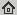# Non-smooth Non-convex Bregman Minimization: Unification and new Algorithms

Technical Report , arXiv:1707.02278 [math.OC], 2017
Abstract: We propose a unifying algorithm for non-smooth non-convex optimization. The algorithm approximates the objective function by a convex model function and finds an approximate (Bregman) proximal point of the convex model. This approximate minimizer of the model function yields a descent direction, along which the next iterate is found. Complemented with an Armijo-like line search strategy, we obtain a flexible algorithm for which we prove (subsequential) convergence to a stationary point under weak assumptions on the growth of the model function error. Special instances of the algorithm with a Euclidean distance function are, for example, Gradient Descent, Forward--Backward Splitting, ProxDescent, without the common requirement of a "Lipschitz continuous gradient". In addition, we consider a broad class of Bregman distance functions (generated by Legendre functions) replacing the Euclidean distance. The algorithm has a wide range of applications including many linear and non-linear inverse problems in image processing and machine learning.

## BibTex reference

```@TechReport{OB17b,
author       = "P. Ochs and J. Fadili and T. Brox",
title        = "Non-smooth Non-convex Bregman Minimization: Unification and new Algorithms",
institution  = "arXiv:1707.02278 [math.OC]",
month        = " ",
year         = "2017",
url          = "http://lmb.informatik.uni-freiburg.de/Publications/2017/OB17b"
}
```